# Frank Solutions for Class 9 Maths Chapter 16 Similarity

Frank Solutions for Class 9 Maths Chapter 16 Similarity provides students, accurate answers to build a strong foundation in the next academic year. Students are suggested to have access to a reliable resource in order to understand the concepts and make learning hassle-free. The main purpose of preparing solutions is to make learning fun and interesting among students. Regular practice of these solutions lessens the strain on students in examinations. For more conceptual knowledge, students can make use of Frank Solutions for Class 9 Maths Chapter 16 Similarity PDF, from the below provided links

Chapter 16 contains answers with simple step by step explanations. These solutions boost confidence among students to attempt the exam. The solutions for each and every question are 100% accurate and are explained elaborately clearing the confusion while solving textbook problems.

## Frank Solutions for Class 9 Maths Chapter 16 Similarity Download PDF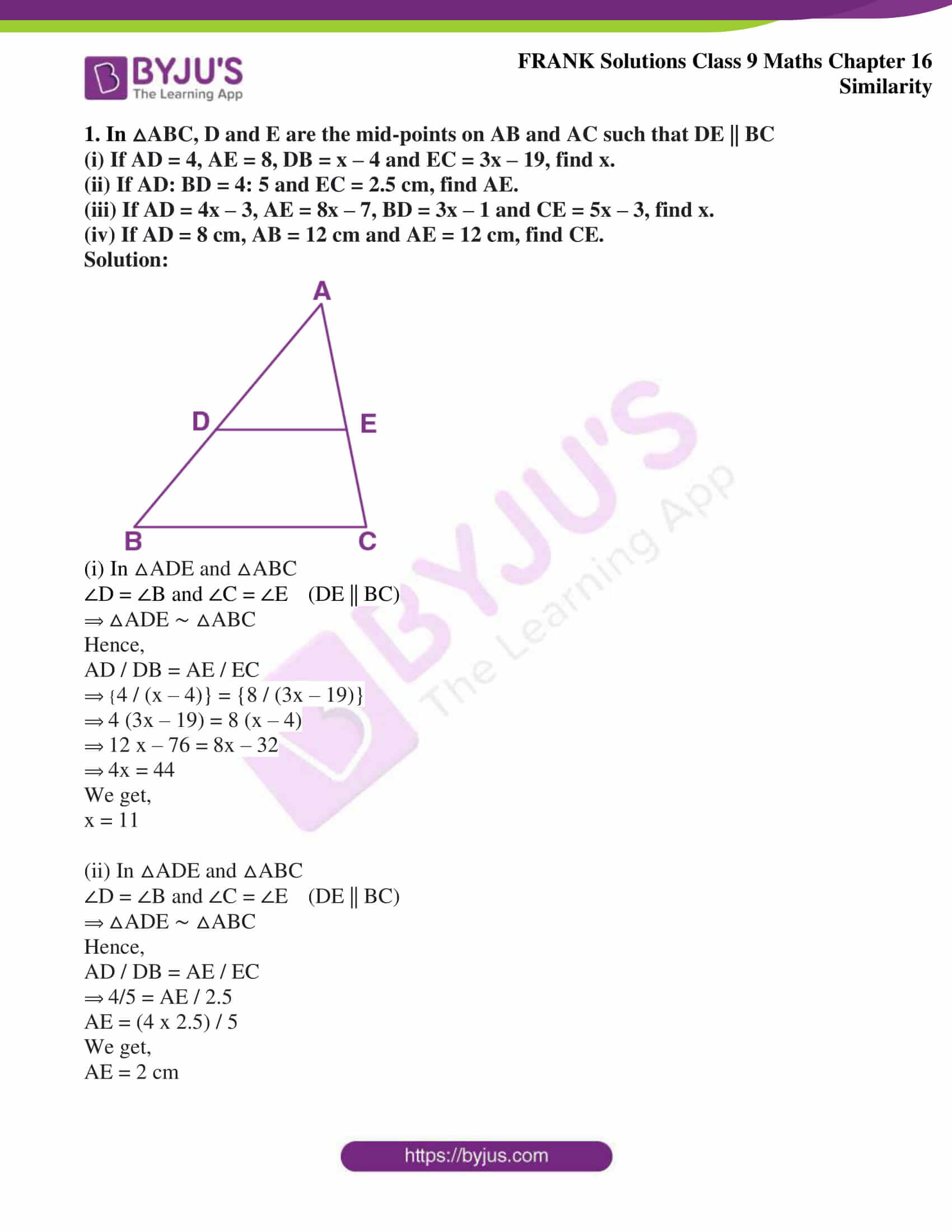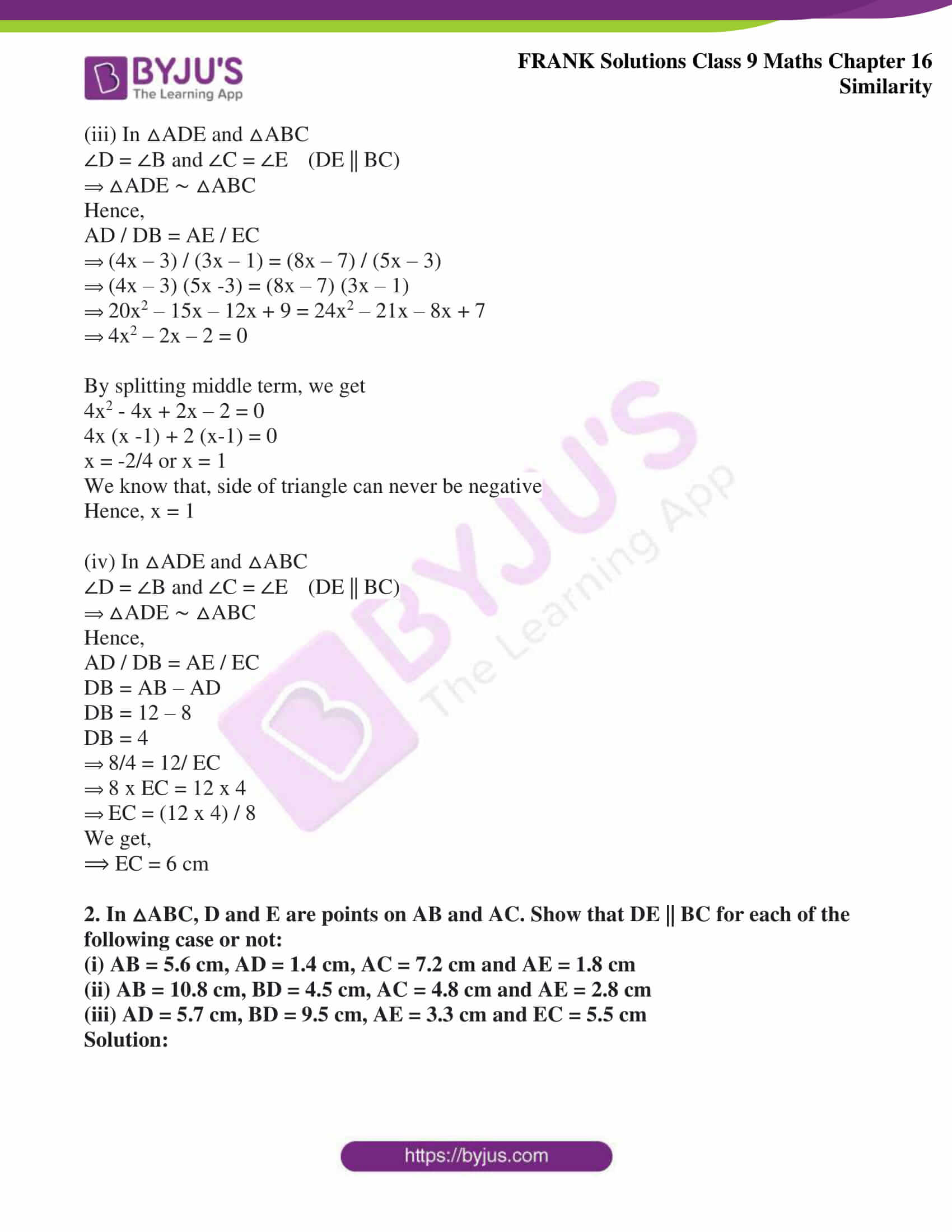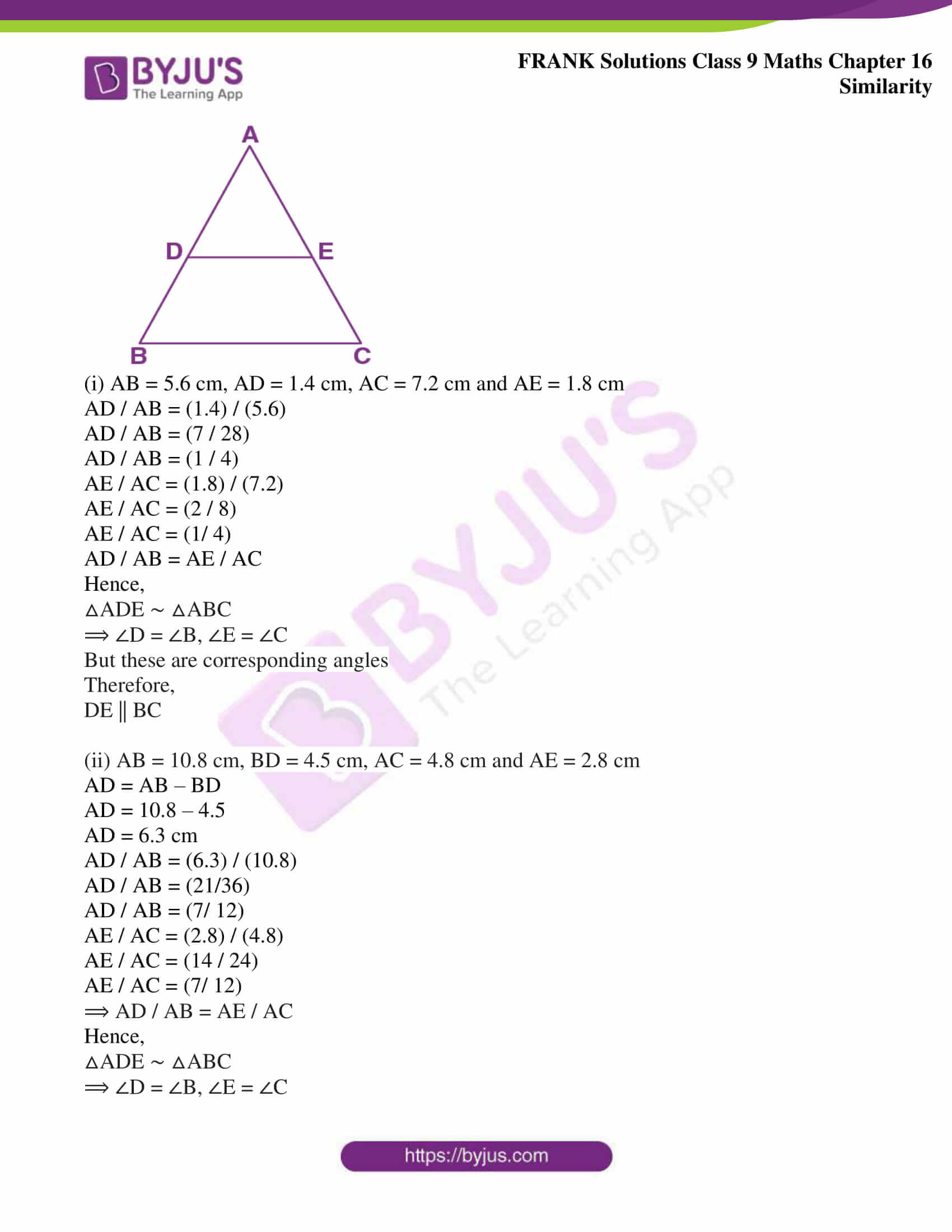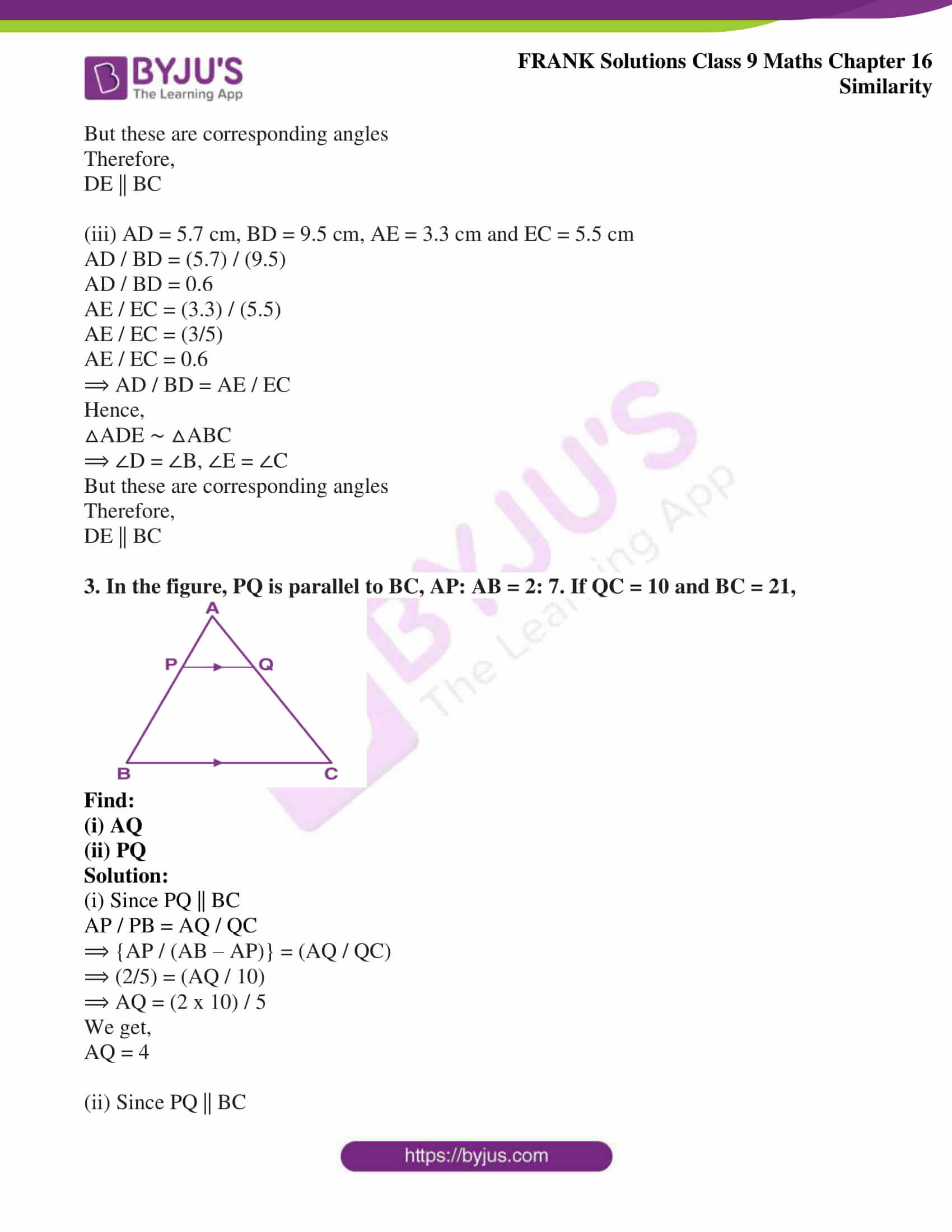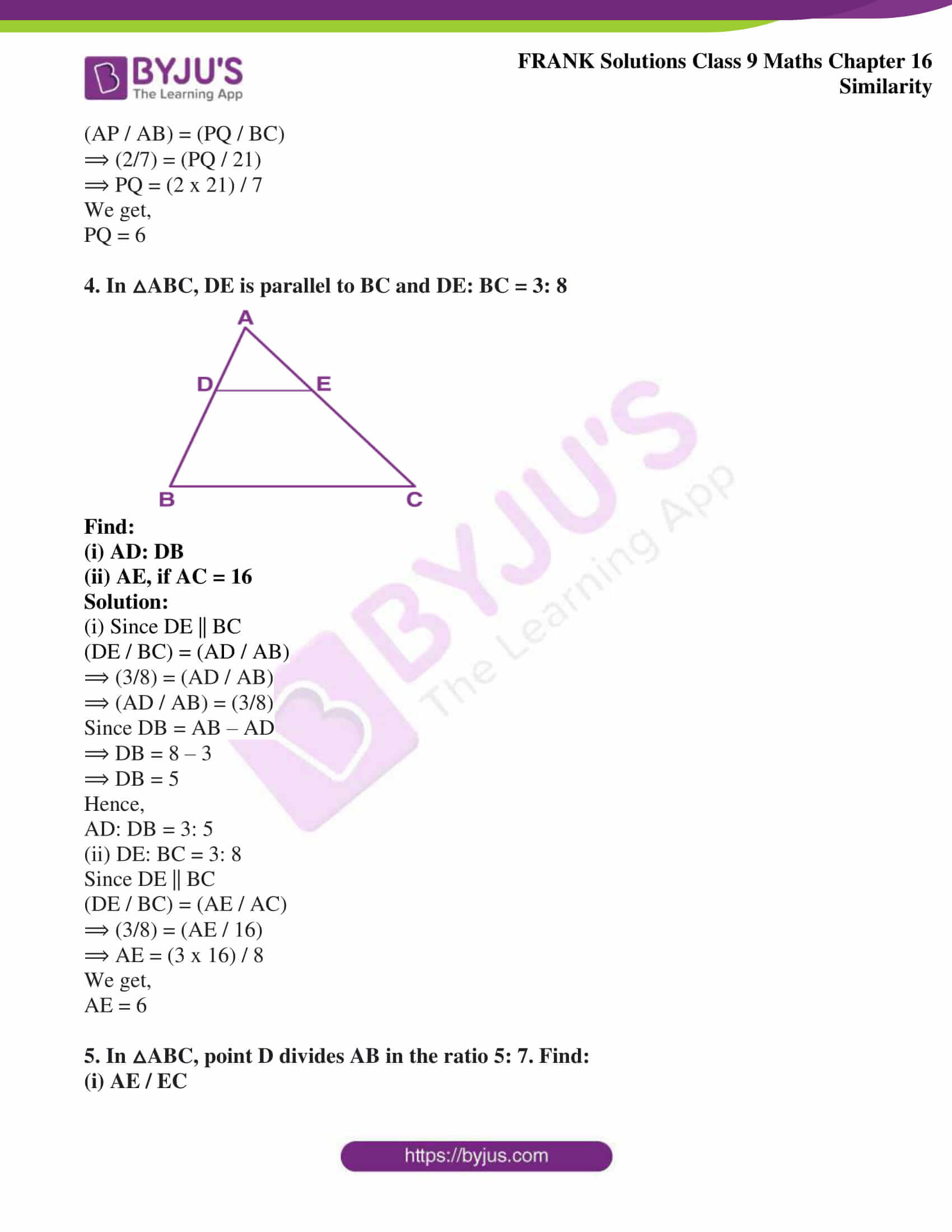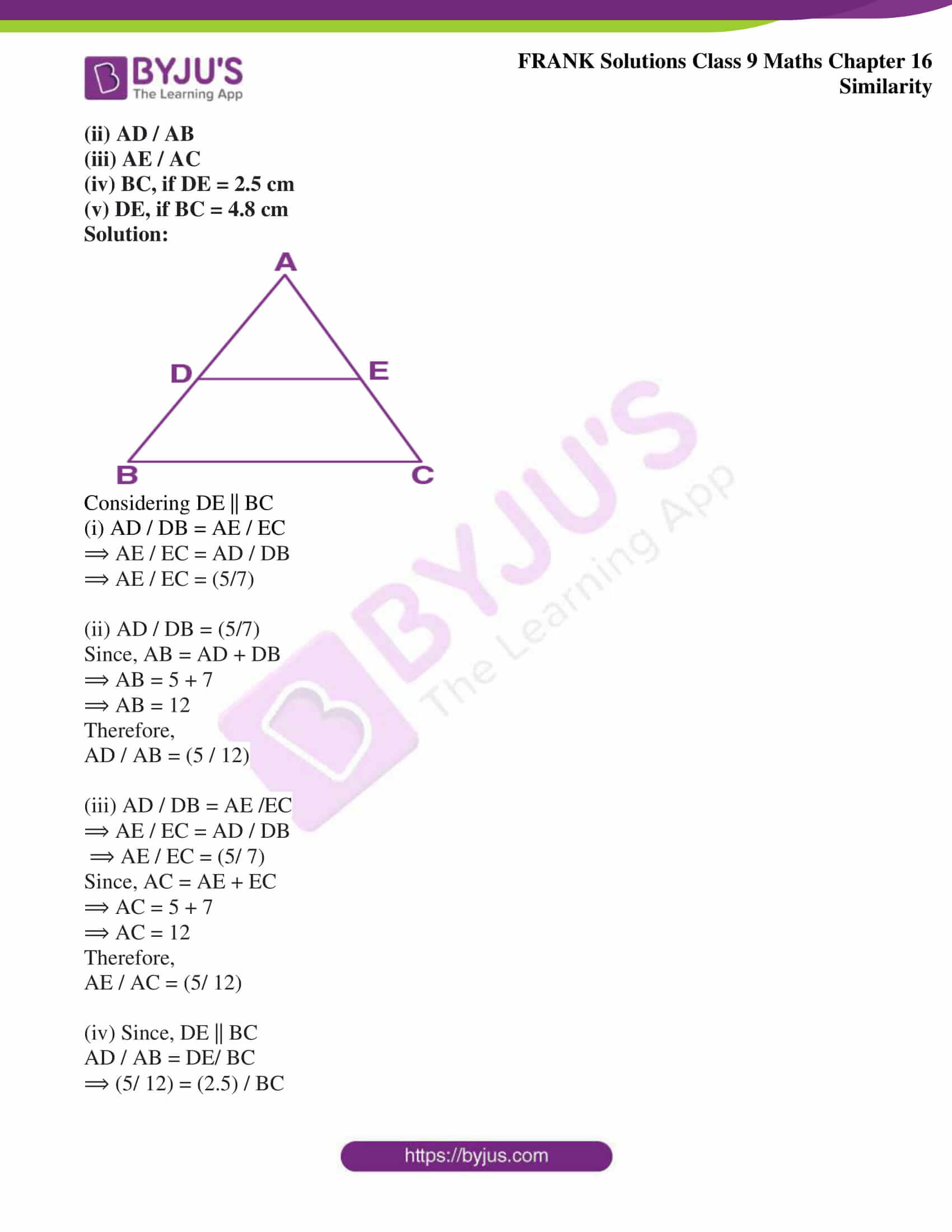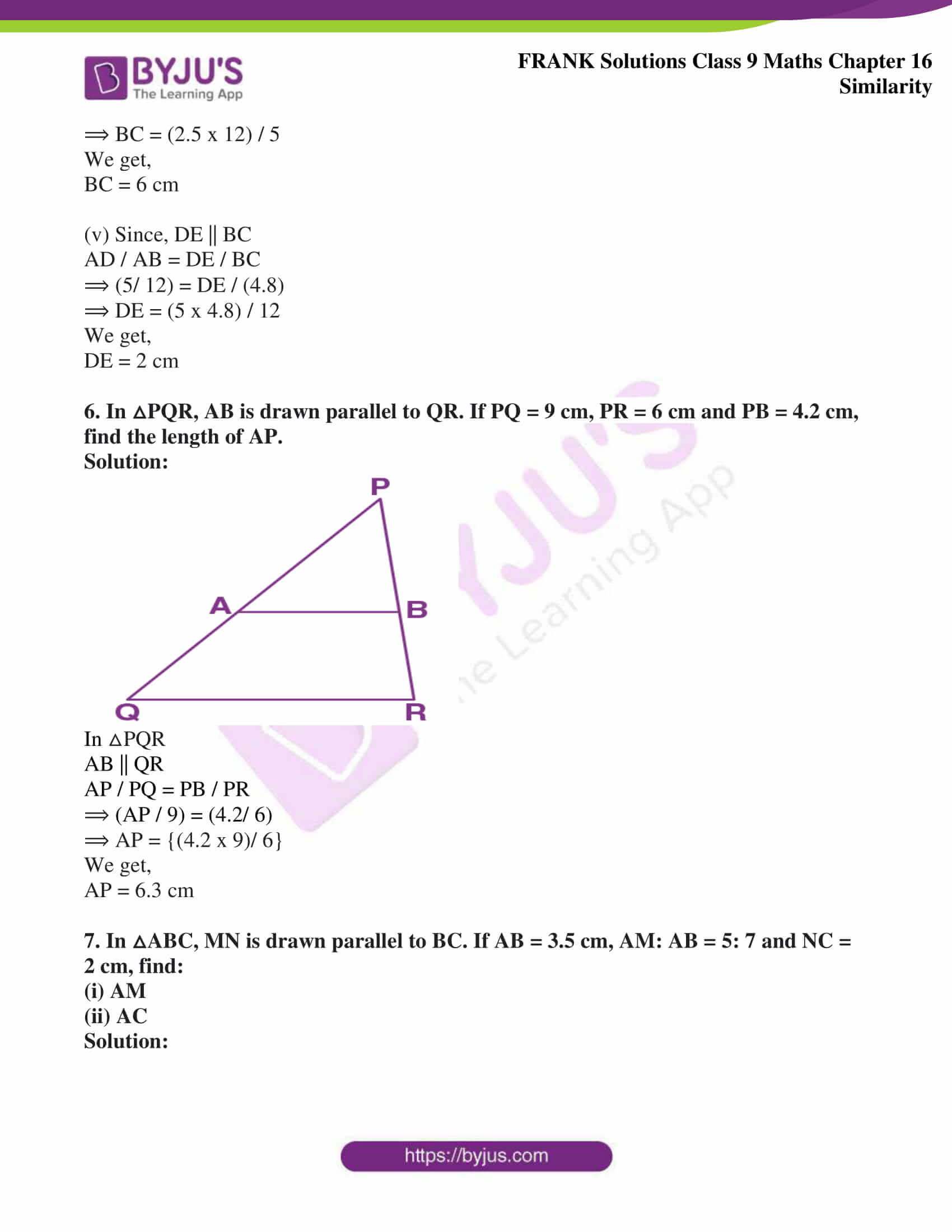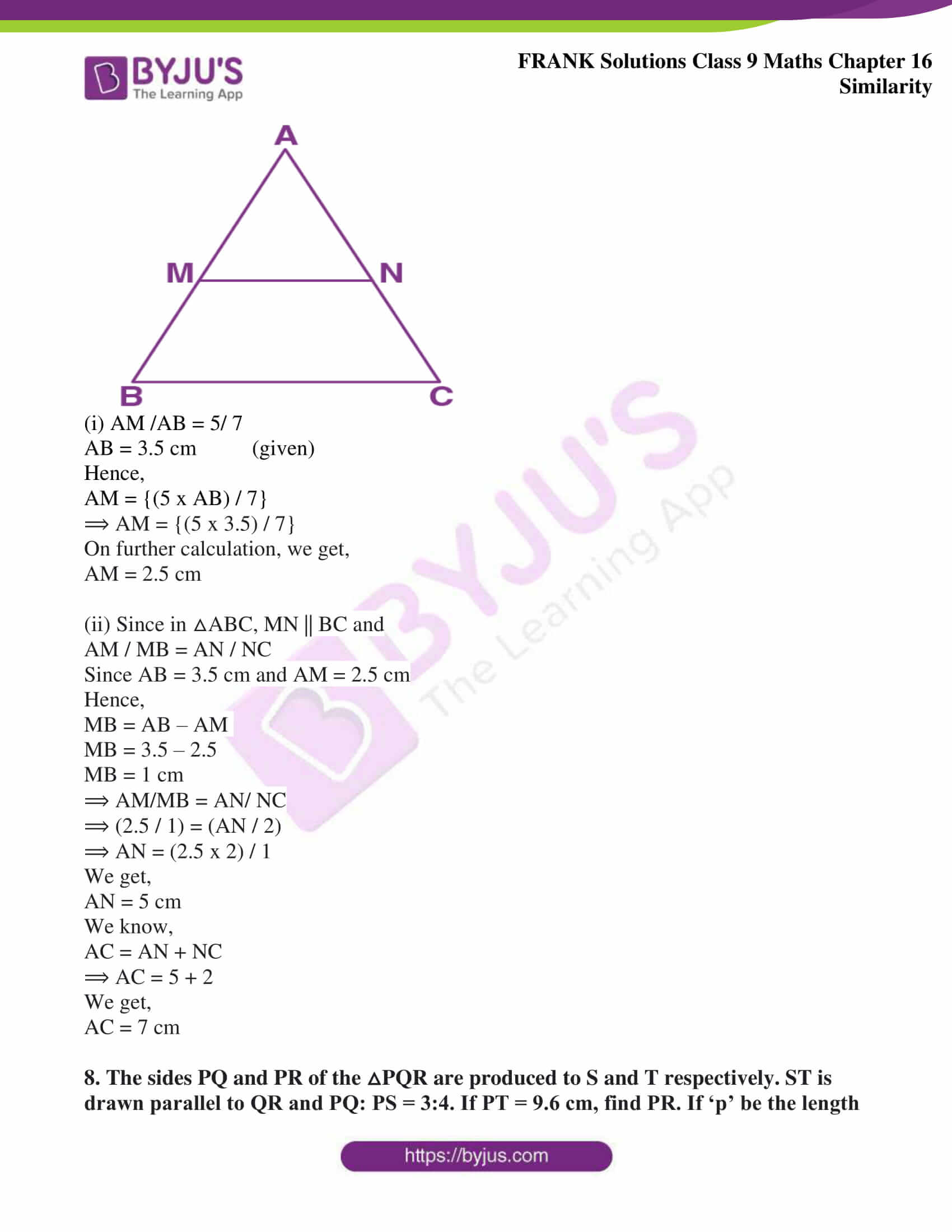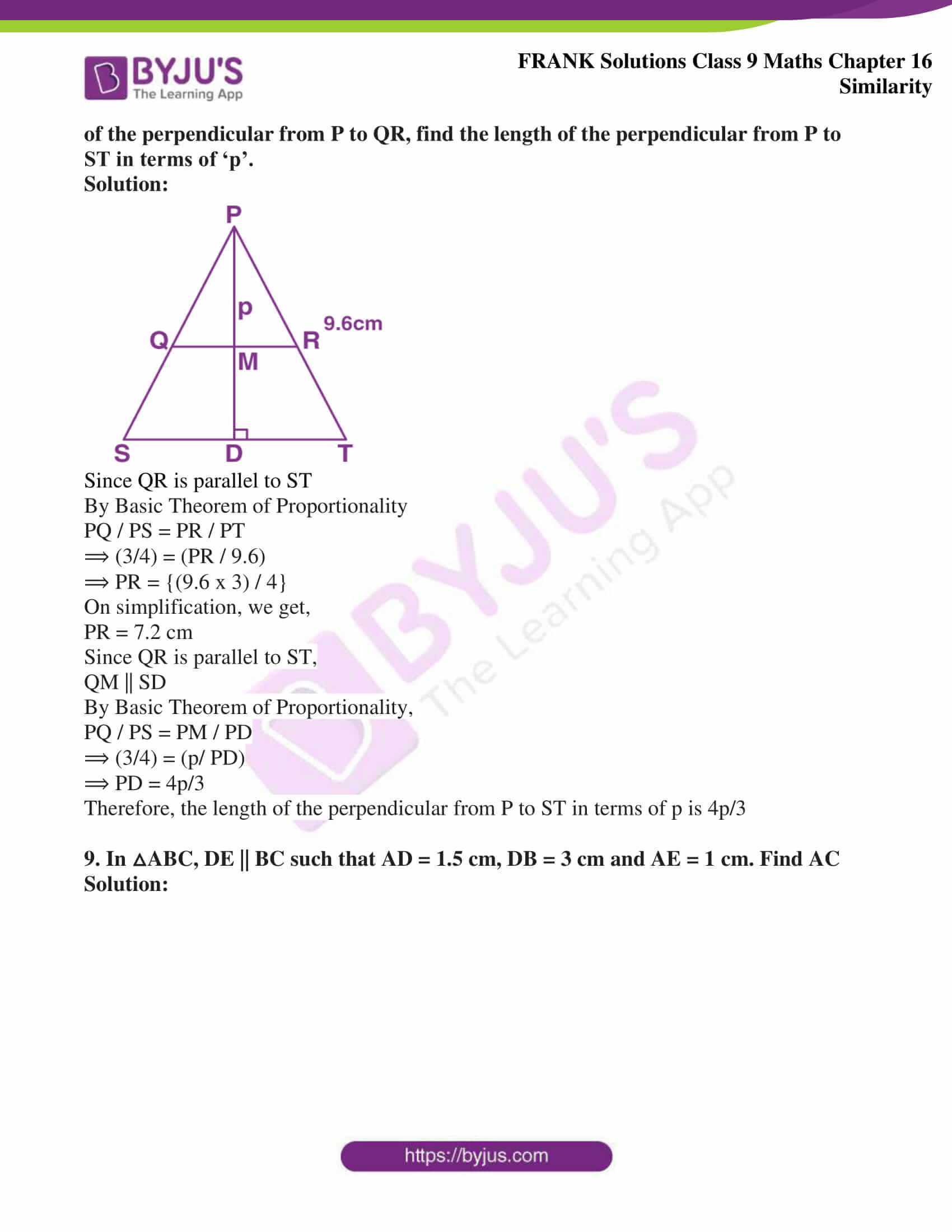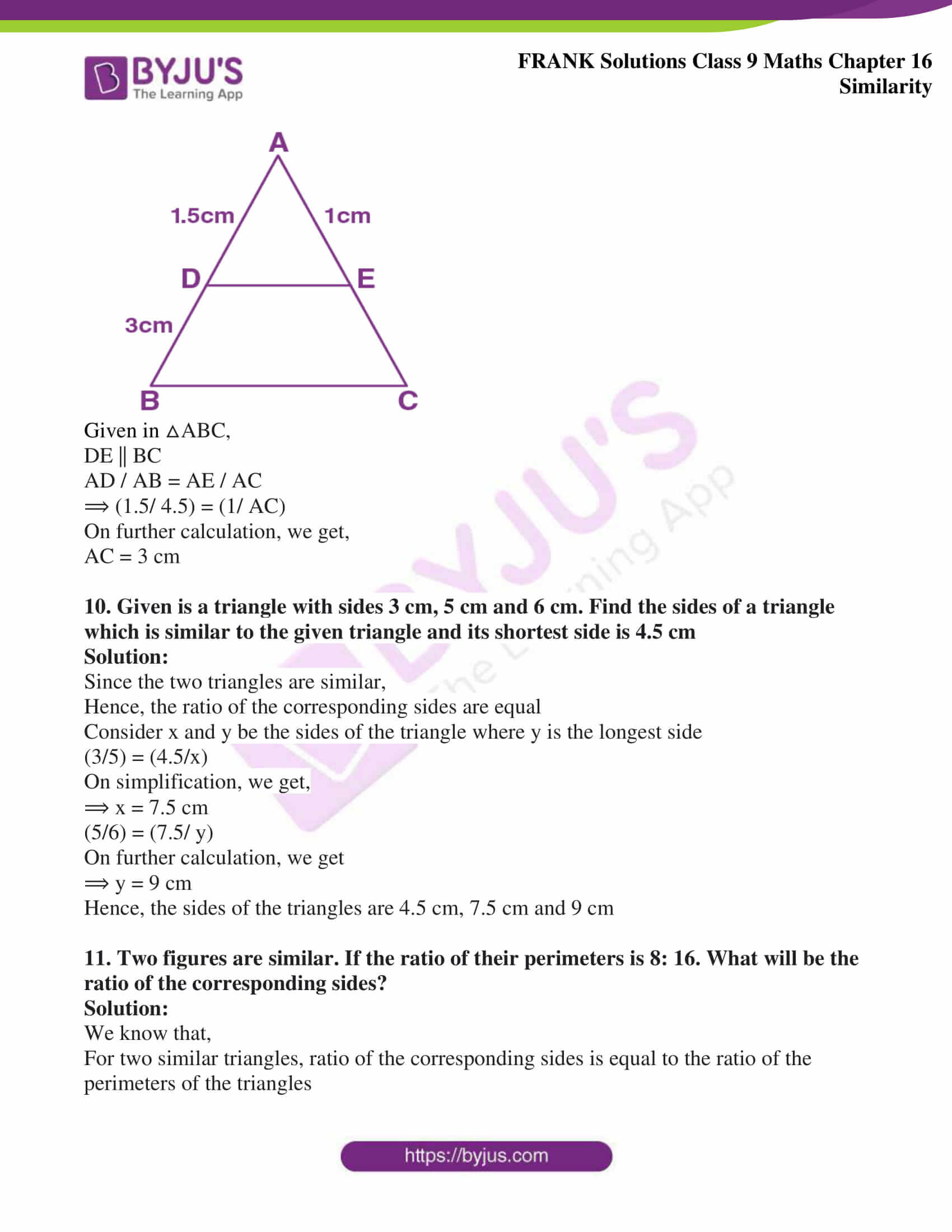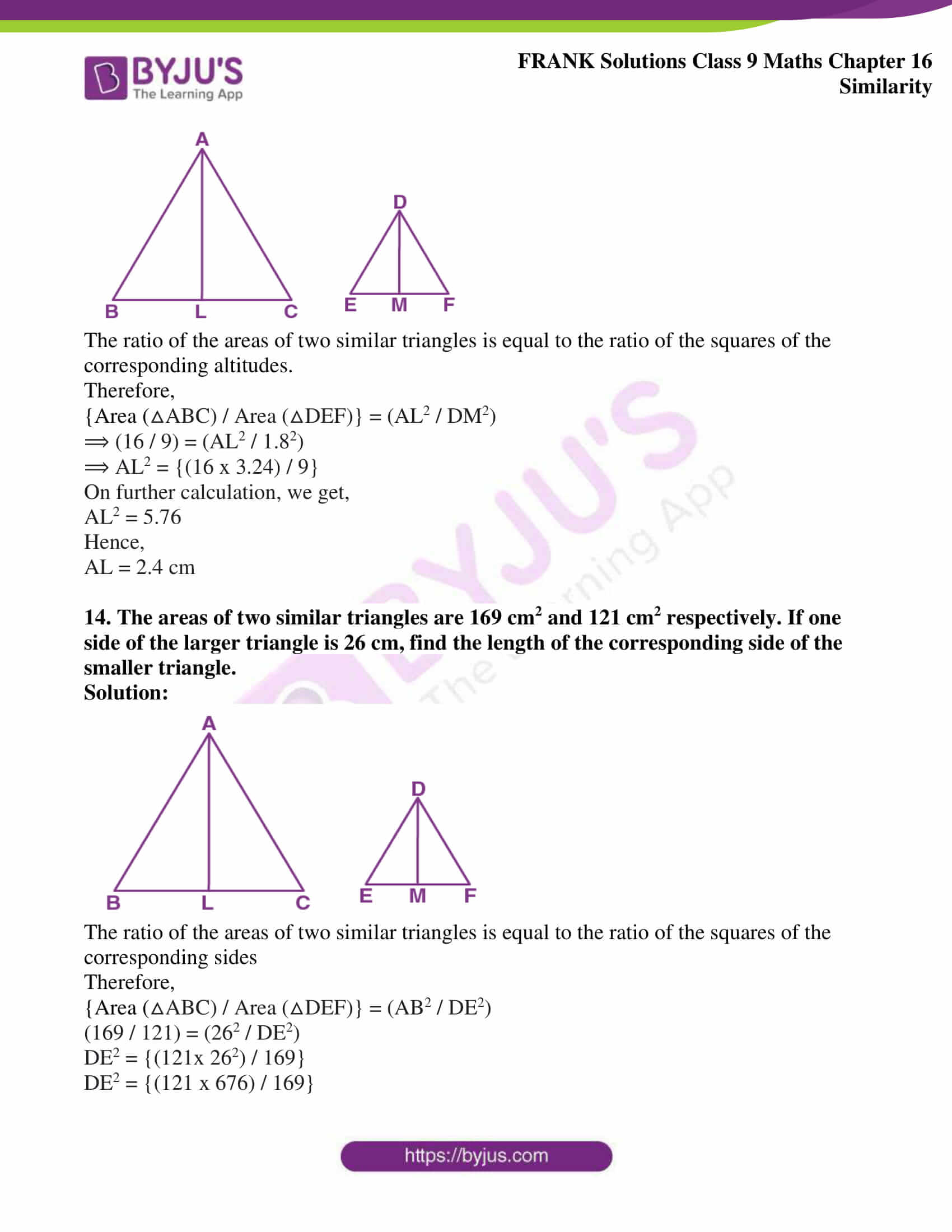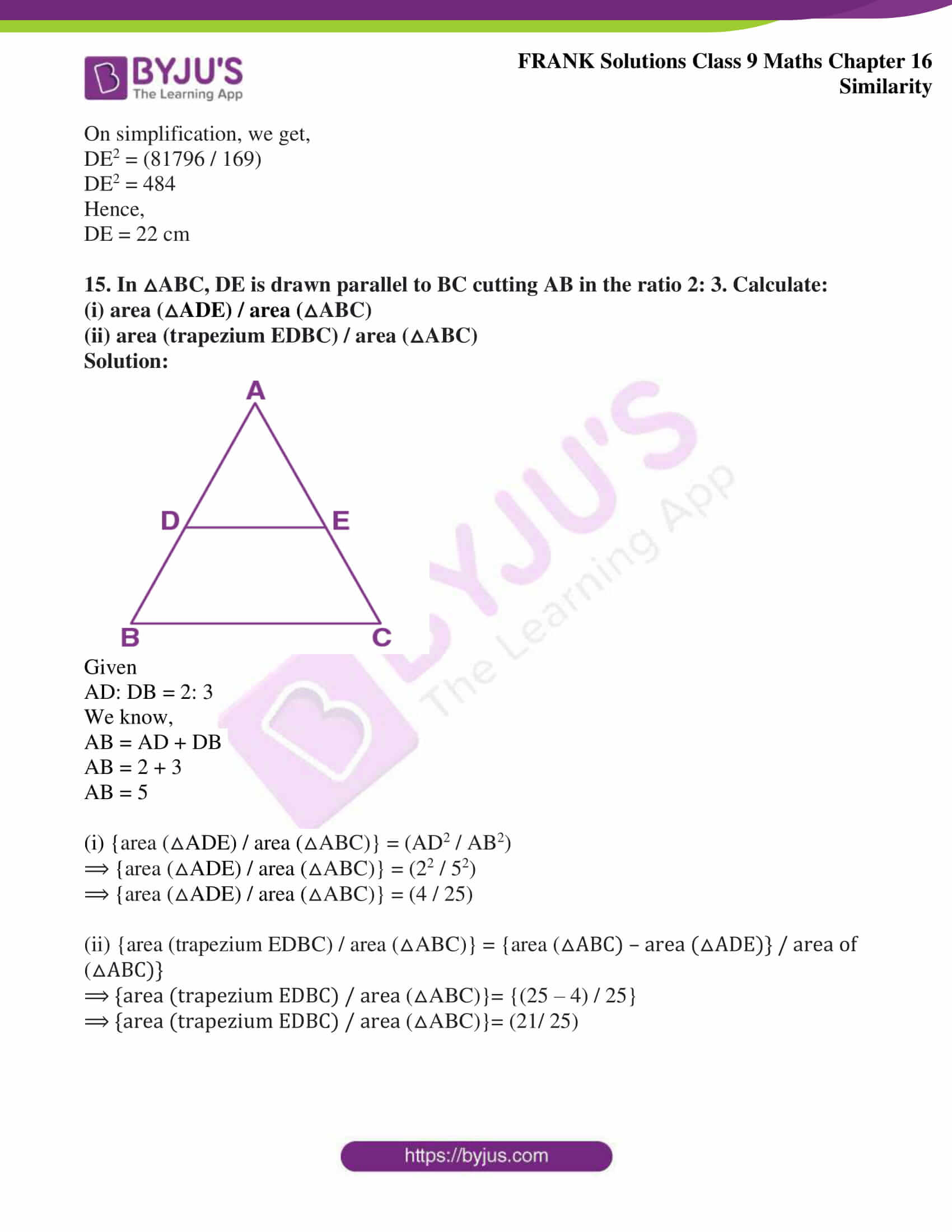## Access Frank Solutions for Class 9 Maths Chapter 16 Similarity

1. In △ABC, D and E are the mid-points on AB and AC such that DE || BC

(i) If AD = 4, AE = 8, DB = x – 4 and EC = 3x – 19, find x.

(ii) If AD: BD = 4: 5 and EC = 2.5 cm, find AE.

(iii) If AD = 4x – 3, AE = 8x – 7, BD = 3x – 1 and CE = 5x – 3, find x.

(iv) If AD = 8 cm, AB = 12 cm and AE = 12 cm, find CE.

Solution: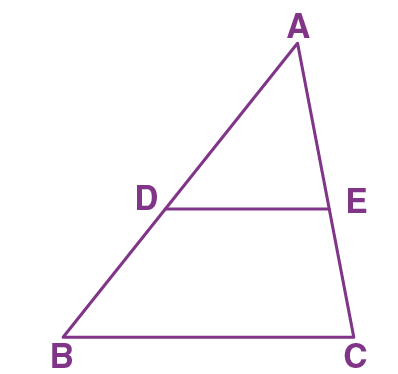∠D = ∠B and ∠C = ∠E (DE || BC)

Hence,

AD / DB = AE / EC

⟹ {4 / (x – 4)} = {8 / (3x – 19)}

⟹ 4 (3x – 19) = 8 (x – 4)

⟹ 12 x – 76 = 8x – 32

⟹ 4x = 44

We get,

x = 11

∠D = ∠B and ∠C = ∠E (DE || BC)

Hence,

AD / DB = AE / EC

⟹ 4/5 = AE / 2.5

AE = (4 x 2.5) / 5

We get,

AE = 2 cm

∠D = ∠B and ∠C = ∠E (DE || BC)

Hence,

AD / DB = AE / EC

⟹ (4x – 3) / (3x – 1) = (8x – 7) / (5x – 3)

⟹ (4x – 3) (5x -3) = (8x – 7) (3x – 1)

⟹ 20x2 – 15x – 12x + 9 = 24x2 – 21x – 8x + 7

⟹ 4x2 – 2x – 2 = 0

By splitting middle term, we get

4x2 – 4x + 2x – 2 = 0

4x (x -1) + 2 (x-1) = 0

x = -2/4 or x = 1

We know that, side of triangle can never be negative

Hence, x = 1

∠D = ∠B and ∠C = ∠E (DE || BC)

Hence,

AD / DB = AE / EC

DB = 12 – 8

DB = 4

⟹ 8/4 = 12/ EC

⟹ 8 x EC = 12 x 4

⟹ EC = (12 x 4) / 8

We get,

⟹ EC = 6 cm

2. In △ABC, D and E are points on AB and AC. Show that DE || BC for each of the following case or not:

(i) AB = 5.6 cm, AD = 1.4 cm, AC = 7.2 cm and AE = 1.8 cm

(ii) AB = 10.8 cm, BD = 4.5 cm, AC = 4.8 cm and AE = 2.8 cm

(iii) AD = 5.7 cm, BD = 9.5 cm, AE = 3.3 cm and EC = 5.5 cm

Solution: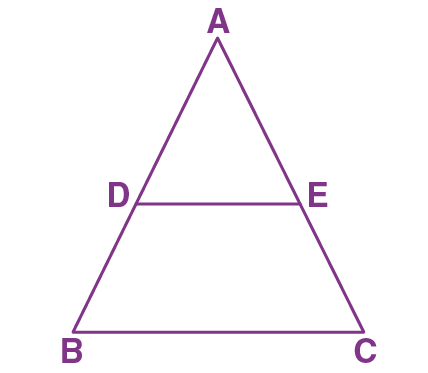(i) AB = 5.6 cm, AD = 1.4 cm, AC = 7.2 cm and AE = 1.8 cm

AD / AB = (1.4) / (5.6)

AD / AB = (7 / 28)

AD / AB = (1 / 4)

AE / AC = (1.8) / (7.2)

AE / AC = (2 / 8)

AE / AC = (1/ 4)

AD / AB = AE / AC

Hence,

⟹ ∠D = ∠B, ∠E = ∠C

But these are corresponding angles

Therefore,

DE || BC

(ii) AB = 10.8 cm, BD = 4.5 cm, AC = 4.8 cm and AE = 2.8 cm

AD / AB = (6.3) / (10.8)

AD / AB = (7/ 12)

AE / AC = (2.8) / (4.8)

AE / AC = (14 / 24)

AE / AC = (7/ 12)

⟹ AD / AB = AE / AC

Hence,

⟹ ∠D = ∠B, ∠E = ∠C

But these are corresponding angles

Therefore,

DE || BC

(iii) AD = 5.7 cm, BD = 9.5 cm, AE = 3.3 cm and EC = 5.5 cm

AD / BD = (5.7) / (9.5)

AE / EC = (3.3) / (5.5)

AE / EC = (3/5)

AE / EC = 0.6

⟹ AD / BD = AE / EC

Hence,

⟹ ∠D = ∠B, ∠E = ∠C

But these are corresponding angles

Therefore,

DE || BC

3. In the figure, PQ is parallel to BC, AP: AB = 2: 7. If QC = 10 and BC = 21,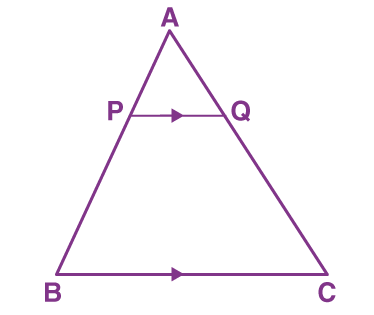Find:

(i) AQ

(ii) PQ

Solution:

(i) Since PQ || BC

AP / PB = AQ / QC

⟹ {AP / (AB – AP)} = (AQ / QC)

⟹ (2/5) = (AQ / 10)

⟹ AQ = (2 x 10) / 5

We get,

AQ = 4

(ii) Since PQ || BC

(AP / AB) = (PQ / BC)

⟹ (2/7) = (PQ / 21)

⟹ PQ = (2 x 21) / 7

We get,

PQ = 6

4. In △ABC, DE is parallel to BC and DE: BC = 3: 8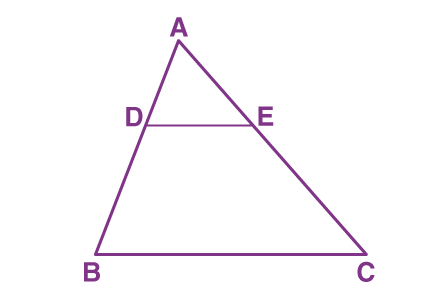Find:

(ii) AE, if AC = 16

Solution:

(i) Since DE || BC

(DE / BC) = (AD / AB)

⟹ (3/8) = (AD / AB)

⟹ (AD / AB) = (3/8)

Since DB = AB – AD

⟹ DB = 8 – 3

⟹ DB = 5

Hence,

(ii) DE: BC = 3: 8

Since DE || BC

(DE / BC) = (AE / AC)

⟹ (3/8) = (AE / 16)

⟹ AE = (3 x 16) / 8

We get,

AE = 6

5. In △ABC, point D divides AB in the ratio 5: 7. Find:

(i) AE / EC

(iii) AE / AC

(iv) BC, if DE = 2.5 cm

(v) DE, if BC = 4.8 cm

Solution: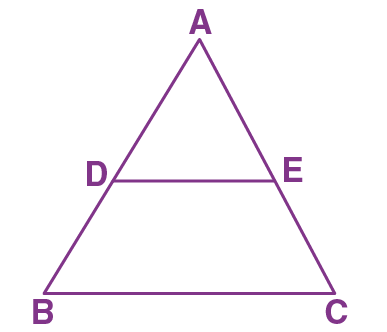Considering DE || BC

(i) AD / DB = AE / EC

⟹ AE / EC = AD / DB

⟹ AE / EC = (5/7)

(ii) AD / DB = (5/7)

Since, AB = AD + DB

⟹ AB = 5 + 7

⟹ AB = 12

Therefore,

AD / AB = (5 / 12)

(iii) AD / DB = AE /EC

⟹ AE / EC = AD / DB

⟹ AE / EC = (5/ 7)

Since, AC = AE + EC

⟹ AC = 5 + 7

⟹ AC = 12

Therefore,

AE / AC = (5/ 12)

(iv) Since, DE || BC

AD / AB = DE/ BC

⟹ (5/ 12) = (2.5) / BC

⟹ BC = (2.5 x 12) / 5

We get,

BC = 6 cm

(v) Since, DE || BC

AD / AB = DE / BC

⟹ (5/ 12) = DE / (4.8)

⟹ DE = (5 x 4.8) / 12

We get,

DE = 2 cm

6. In △PQR, AB is drawn parallel to QR. If PQ = 9 cm, PR = 6 cm and PB = 4.2 cm, find the length of AP.

Solution: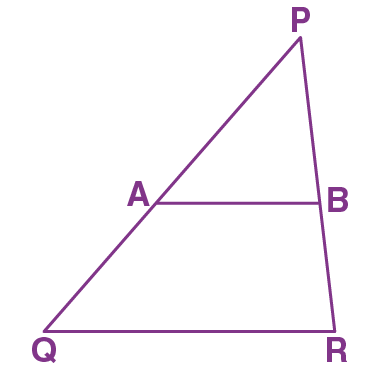In △PQR

AB || QR

AP / PQ = PB / PR

⟹ (AP / 9) = (4.2/ 6)

⟹ AP = {(4.2 x 9)/ 6}

We get,

AP = 6.3 cm

7. In △ABC, MN is drawn parallel to BC. If AB = 3.5 cm, AM: AB = 5: 7 and NC = 2 cm, find:

(i) AM

(ii) AC

Solution: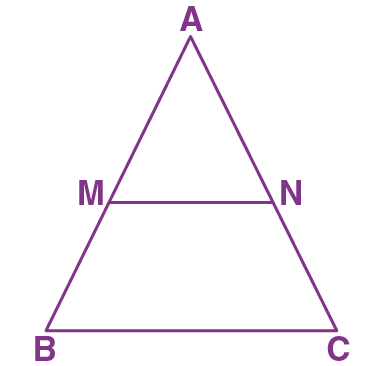(i) AM /AB = 5/ 7

AB = 3.5 cm (given)

Hence,

AM = {(5 x AB) / 7}

⟹ AM = {(5 x 3.5) / 7}

On further calculation, we get,

AM = 2.5 cm

(ii) Since in △ABC, MN || BC and

AM / MB = AN / NC

Since AB = 3.5 cm and AM = 2.5 cm

Hence,

MB = AB – AM

MB = 3.5 – 2.5

MB = 1 cm

⟹ AM/MB = AN/ NC

⟹ (2.5 / 1) = (AN / 2)

⟹ AN = (2.5 x 2) / 1

We get,

AN = 5 cm

We know,

AC = AN + NC

⟹ AC = 5 + 2

We get,

AC = 7 cm

8. The sides PQ and PR of the △PQR are produced to S and T respectively. ST is drawn parallel to QR and PQ: PS = 3:4. If PT = 9.6 cm, find PR. If ‘p’ be the length of the perpendicular from P to QR, find the length of the perpendicular from P to ST in terms of ‘p’.

Solution: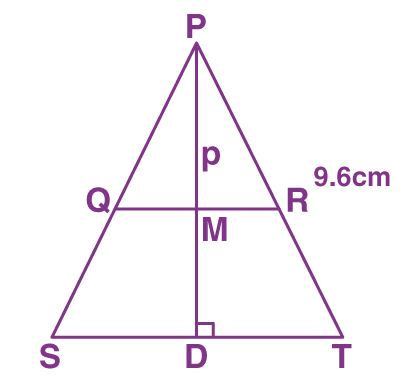Since QR is parallel to ST

By Basic Theorem of Proportionality

PQ / PS = PR / PT

⟹ (3/4) = (PR / 9.6)

⟹ PR = {(9.6 x 3) / 4}

On simplification, we get,

PR = 7.2 cm

Since QR is parallel to ST,

QM || SD

By Basic Theorem of Proportionality,

PQ / PS = PM / PD

⟹ (3/4) = (p/ PD)

⟹ PD = 4p/3

Therefore, the length of the perpendicular from P to ST in terms of p is 4p/3

9. In △ABC, DE || BC such that AD = 1.5 cm, DB = 3 cm and AE = 1 cm. Find AC

Solution: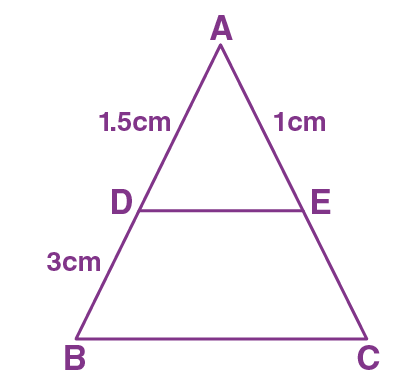Given in △ABC,

DE || BC

AD / AB = AE / AC

⟹ (1.5/ 4.5) = (1/ AC)

On further calculation, we get,

AC = 3 cm

10. Given is a triangle with sides 3 cm, 5 cm and 6 cm. Find the sides of a triangle which is similar to the given triangle and its shortest side is 4.5 cm

Solution:

Since the two triangles are similar,

Hence, the ratio of the corresponding sides are equal

Consider x and y be the sides of the triangle where y is the longest side

(3/5) = (4.5/x)

On simplification, we get,

⟹ x = 7.5 cm

(5/6) = (7.5/ y)

On further calculation, we get

⟹ y = 9 cm

Hence, the sides of the triangles are 4.5 cm, 7.5 cm and 9 cm

11. Two figures are similar. If the ratio of their perimeters is 8: 16. What will be the ratio of the corresponding sides?

Solution:

We know that,

For two similar triangles, ratio of the corresponding sides is equal to the ratio of the perimeters of the triangles

Hence,

Ratio of the corresponding sides = (8/16) = (1/2)

That is, ratio of the corresponding sides is 1:2

12. Harmeet is 6 feet tall and casts a shadow of 3 feet long. What is the height of a nearby pole if it casts a shadow of 12 feet long at the same time?

Solution: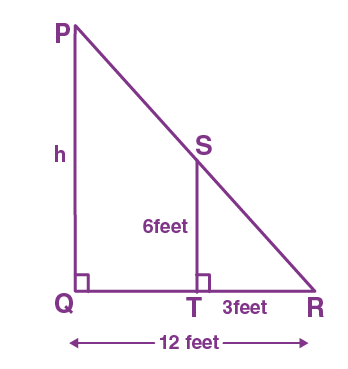Harmeet and the pole will be perpendicular to the ground

So,

PQ || ST

In △PQR and △STR,

∠PQR = ∠STR …… (Both are right angles)

∠PRQ = ∠SRT…….. (common angle)

△PQR ∼ △STR ……. (AA criterion for similarity)

PQ / ST = QR / TR

⟹ (h/6) = (12/ 3)

On simplification, we get,

h = 24 feet

Therefore, the height of the pole is 24 feet

13. The areas of two similar triangles are 16 cm2 and 9 cm2 respectively. If the altitude of the smaller triangle is 1.8 cm, find the length of the altitude corresponding to the larger triangle.

Solution: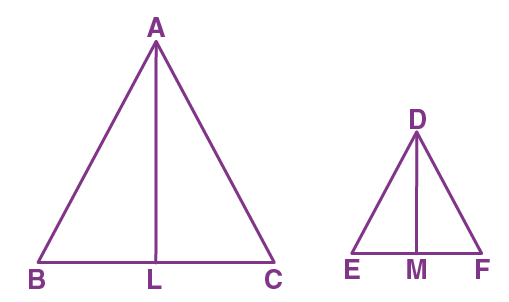The ratio of the areas of two similar triangles is equal to the ratio of the squares of the corresponding altitudes.

Therefore,

{Area (△ABC) / Area (△DEF)} = (AL2 / DM2)

⟹ (16 / 9) = (AL2 / 1.82)

⟹ AL2 = {(16 x 3.24) / 9}

On further calculation, we get,

AL2 = 5.76

Hence,

AL = 2.4 cm

14. The areas of two similar triangles are 169 cm2 and 121 cm2 respectively. If one side of the larger triangle is 26 cm, find the length of the corresponding side of the smaller triangle.

Solution:The ratio of the areas of two similar triangles is equal to the ratio of the squares of the corresponding sides

Therefore,

{Area (△ABC) / Area (△DEF)} = (AB2 / DE2)

(169 / 121) = (262 / DE2)

DE2 = {(121x 262) / 169}

DE2 = {(121 x 676) / 169}

On simplification, we get,

DE2 = (81796 / 169)

DE2 = 484

Hence,

DE = 22 cm

15. In △ABC, DE is drawn parallel to BC cutting AB in the ratio 2: 3. Calculate:

(i) area (△ADE) / area (△ABC)

(ii) area (trapezium EDBC) / area (△ABC)

Solution: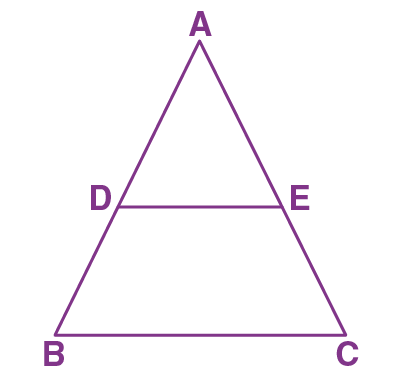Given

We know,

AB = 2 + 3

AB = 5